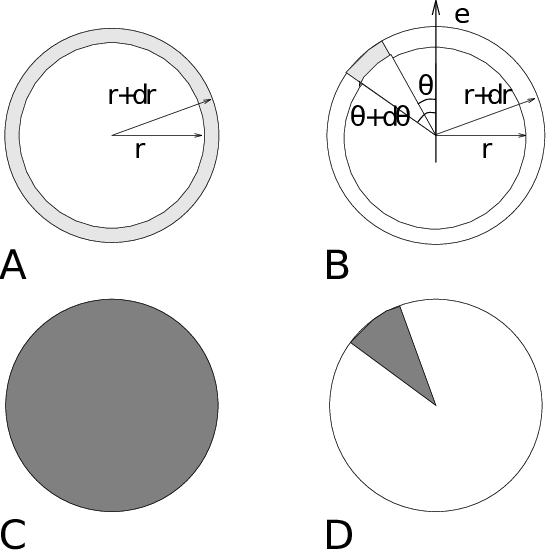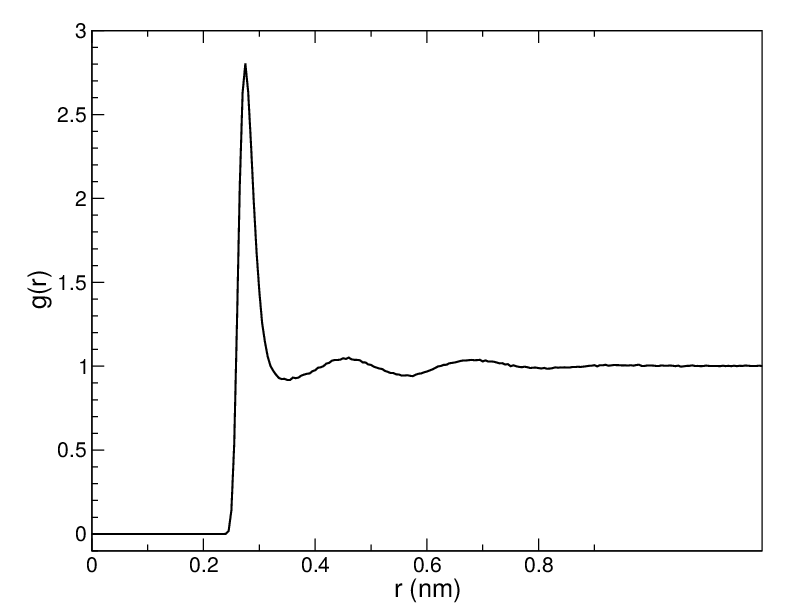The radial distribution function (RDF) or pair correlation function $$g_{AB}(r)$$ between particles of type $$A$$ and $$B$$ is defined in the following way:
(1)$\begin{split}\begin{array}{rcl} g_{AB}(r)&=& \frac{\langle \rho_B(r) \rangle}{\langle\rho_B\rangle_{local}}} \\ &=& \frac{1}{\langle\rho_B\rangle_{local}}}\frac{1}{N_A}} \sum_{i \in A}^{N_A} \sum_{j \in B}^{N_B} \frac{\delta( r_{ij} - r )}{4 \pi r^2}} \\ \end{array}\end{split$

with $$\langle\rho_B(r)\rangle$$ the particle density of type $$B$$ at a distance $$r$$ around particles $$A$$, and $$\langle\rho_B\rangle_{local}$$ the particle density of type $$B$$ averaged over all spheres around particles $$A$$ with radius $$r_{max}$$ (see Fig. 51 C).Fig. 51 Definition of slices in gmx rdf: A. $$g_{AB}(r)$$. B. $$g_{AB}(r,\theta)$$. The slices are colored gray. C. Normalization $$\langle\rho_B\rangle_{local}$$. D. Normalization $$\langle\rho_B\rangle_{local,\:\theta }$$. Normalization volumes are colored gray.

Usually the value of $$r_{max}$$ is half of the box length. The averaging is also performed in time. In practice the analysis program gmx rdf divides the system into spherical slices (from $$r$$ to $$r+dr$$, see Fig. 51 A) and makes a histogram in stead of the $$\delta$$-function. An example of the RDF of oxygen-oxygen in SPC water :ref:80 is given in Fig. 52Fig. 52 $$g_{OO}(r)$$ for Oxygen-Oxygen of SPC-water.

With gmx rdf it is also possible to calculate an angle dependent rdf $$g_{AB}(r,\theta)$$, where the angle $$\theta$$ is defined with respect to a certain laboratory axis $${\bf e}$$, see Fig. 51 B.

(2)$g_{AB}(r,\theta) = {1 \over \langle\rho_B\rangle_{local,\:\theta }} {1 \over N_A} \sum_{i \in A}^{N_A} \sum_{j \in B}^{N_B} {\delta( r_{ij} - r ) \delta(\theta_{ij} -\theta) \over 2 \pi r^2 sin(\theta)}$
(3)$cos(\theta_{ij}) = {{\bf r}_{ij} \cdot {\bf e} \over \|r_{ij}\| \;\| e\| }$

This $$g_{AB}(r,\theta)$$ is useful for analyzing anisotropic systems. Note that in this case the normalization $$\langle\rho_B\rangle_{local,\:\theta}$$ is the average density in all angle slices from $$\theta$$ to $$\theta + d\theta$$ up to $$r_{max}$$, so angle dependent, see Fig. 51 D.